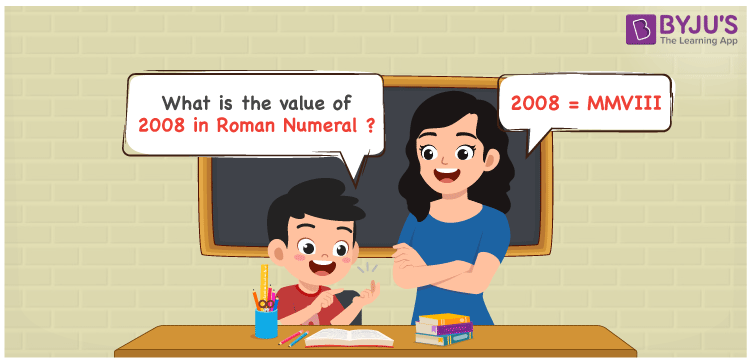# 2008 in Roman Numerals

2008 in Roman Numerals is MMVIII. Any Roman numeral uses only the 7 primary symbols which are I, V, X, L, C, D, and M where M represents 1000, V represents 5 and I represents 1. MMVIII follows the addition rule of Roman numerals and it is the number 2008. What are Roman numerals? can be referred for more details. The details on how to write 2008 is given in the next section of this article.

 Number Roman Numeral 2008 MMVIII

## How to Write 2008 in Roman Numerals?To convert 2008 in Roman Numerals, we need to represent 2008 as the sum of the fundamental symbols. 2008 can be broken down as the sum of 1000,1000, 5 and three 1. Since M is the symbol for 1000, 2000 will be MM, Similarly, 8 will be VIII.

2008 = 1000 + 1000 + 5 + 1 + 1 + 1

2008 = M + M + V + I + I + I

2008 = MMVIII.

## Video Lesson on Roman Numerals## Frequently Asked Questions on 2008 in Roman Numerals

Q1

### How to write the number 2008 in Roman Numerals?

2008 in Roman Numerals is written as MMVIII.
Q2

### Does MMVIII represent Roman Numerals?

Yes. MMVIII represents Roman Numerals and it is the number 2008
Q3

### What is the year 2008 in Roman numerals?

The year 2008 in Roman Numerals is MMVIII.